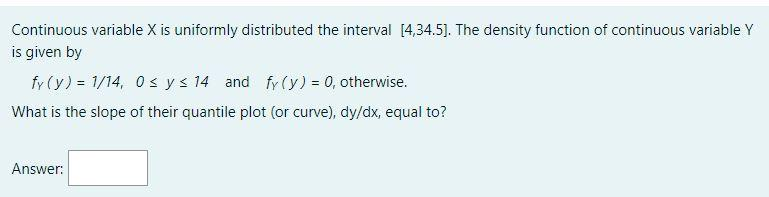# Question Continuous variable $$\mathrm{X}$$ is uniformly distributed the interval $$[4,34.5]$$. The density function of continuous variable $$\mathrm{Y}$$ is given by $f_{Y}(y)=1 / 14,0 \leq y \leq 14 \text { and } f_{Y}(y)=0 \text {, otherwise. }$ What is the slope of their quantile plot (or curve), $$d y / d x$$, equal to? Answer:DDJT9Y The Asker · Probability and StatisticsTranscribed Image Text: Continuous variable $$\mathrm{X}$$ is uniformly distributed the interval $$[4,34.5]$$. The density function of continuous variable $$\mathrm{Y}$$ is given by $f_{Y}(y)=1 / 14,0 \leq y \leq 14 \text { and } f_{Y}(y)=0 \text {, otherwise. }$ What is the slope of their quantile plot (or curve), $$d y / d x$$, equal to? Answer:
More
Transcribed Image Text: Continuous variable $$\mathrm{X}$$ is uniformly distributed the interval $$[4,34.5]$$. The density function of continuous variable $$\mathrm{Y}$$ is given by $f_{Y}(y)=1 / 14,0 \leq y \leq 14 \text { and } f_{Y}(y)=0 \text {, otherwise. }$ What is the slope of their quantile plot (or curve), $$d y / d x$$, equal to? Answer: